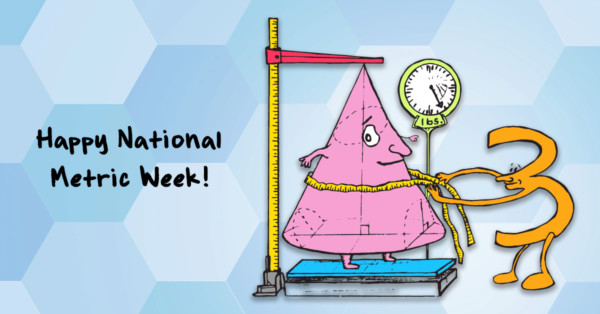* indicates a required field• phone number format invalid• email format invalidProblems detected, please review the form.
Privacy - Terms

Currently doing both In Center and Online Instruction!

# News from Mathnasium of Lead Mine

### Mini Math:National Metric Week

Oct 19, 2016Did you know that every year, the week of October 10 is National Metric Week? There are many great reasons to recognize and celebrate the metric system, the first being its ease of use. Because of this, it’s essentially accepted as the standard measurement system worldwide (with very few countries holding out on full implementation), allowing for consistency in measurement on an unprecedented scale. Subsequently, the metric system has been instrumental in facilitating global trade and other collaborations across nations! The world as we know it would be very different today were it not for the metric system.

The week of October 10 (10/10) is a fitting window of time for National Metric Week, considering that every time you count 10 of a given base metric unit, you’ll get 1 of the next unit.

The units of distance in the metric system are:

millimeter = mm (1/1000 of a meter)

centimeter = cm (1/100 of a meter)

decimeter = dm (1/10 of a meter)

meter = m (1)

dekameter = dam (10 meters)

hectometer = hm (100 meters)

kilometer = km (1,000 meters)

Ten of any of these units is equal to one of the next unit up:

10 millimeters = 1 centimeter

10 centimeters = 1 decimeter

10 decimeters = 1 meter

… and so on!

Calculating area or volume? No problem! You can convert square and cubic units similarly. Square units measure area, calculated by multiplying length × width in many cases. Both the length and width of a given area each change by a factor of 10 (10 × 10 = 100) as you move from one unit to the next:

100 mm2 = 1 cm2

100 cm= 1 dm2

100 dm2 = 1 m2

… and so on!

This means you move the decimal point two places (100) left or right for each unit down or up from your starting point:

Convert 276 km2 to m2.

When we go from a bigger unit to a smaller unit, we move the decimal point to the right. Because “square kilometers” is three units up from (and a bigger unit than) “square meters,” we move the decimal point six places (3 × 2) to the right:

276 km2 = 276,000,000 m2

In that vein, when we use cubic units to measure volume, we’re looking at length × width × height in many cases. Length, width, and height of a given volume each change by a factor of 10 (10 × 10 × 10 = 1,000) as you move from one unit to the next:

1,000 mm3 = 1 cm3

1,000 cm= 1 dm3

1,000 dm3 = 1 m3

… and so on!

This means you move the decimal point three places (1,000) left or right for each unit down or up from your starting point:

Convert 785 cm3 to km3.

When we go from a smaller unit to a bigger unit, we move the decimal point to the left. Because “cubic centimeters” is five units down from (and a smaller unit than) “cubic kilometers,” we move the decimal point 15 places (5 × 3) to the left:

785 cm2 = 0.000000000000785 km2

As the metric system is a decimal (base 10, “deci” meaning “10”) system, in many cases, you don’t need to calculate to convert … simply move the decimal point left or right! This is why it’s extremely easy to convert between metric units—much easier than with other measurement systems out there!

Metric Facts:

• The metric system is also known as Le Systemè International d’Unités—SI for short!
• The original “meter” was defined as one-tenmillionth of the distance from the equator to the North Pole, measured along the prime meridian. Today, it is defined as 1/299,792,458 of the distance light travels in a vacuum in a second.
• Generally speaking, metric prefixes that indicate units larger than the base (i.e. kilo-, tera-, giga-, deka-) have Greek roots, while prefixes that indicate units smaller than the base unit (centi-, deci-, milli-) come from Latin. (There are exceptions and overlaps, of course.)
• The metric system was officially introduced in France in the 1790s. Over the centuries, most countries worldwide adopted it as the standard measurement system. While most countries use metric units exclusively, others have yet to adopt it fully.
• With many elements of daily life measured in miles, inches, feet, pounds, ounces, quarts, gallons, etc., the U.S. remains the only industrialized nation that has yet to go fully metric. However, it is the established measurement system for certain industries, particularly within STEM fields. Other metric holdouts include Myanmar and Liberia.
• Metrication is the process by which countries go metric!

Itching for more metric history? TED-Ed’s video sums it up nicely:

Did you celebrate National Metric Week? If so, how?